Next: 2.8 Summary of construction Up: 2. Choices in the Previous: 2.6 Transition rule   Contents

Subsections

# 2.7 Reversible CA

The following two sections describe special classes of cellular automata, namely partitioned CA and block-CA. Both classes have been introduced to facilitate construction of reversible CA. Reversibility is an important feature of many physical systems. In a reversible system, the direction of time can be reversed by translating the state of a system (e.g., reversing all velocities) and possibly changing the interaction rules. After this reversal, the system follows a reverse sequence of states, going back to the initial state.

For a CA to be reversible, the global state transition function has to be reversible. For this, it has to be a permutation, so that each global state has exactly one successor and exactly one predecessor. In general, it is very difficult to verify this property for a given CA, especially since it has to be verified for all possible sizes of the CA. In order to facilitate the construction of reversible CA, we can introduce constructions that ensure the reversibility of the global state transition function given some local properties. For general CA this is not possible, since the state transition function uses information from the neighbors (see Figure 2.18), and this information flow is not easily reversible.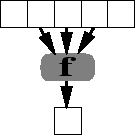## 2.7.1 Partitioned Cellular Automata

The first class of reversible CA avoids the problem of uncontrolled information flow from the neighbors by partitioning the state of a cell into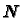parts. Each of these parts is then used by a different neighbor in the updating function, and only one of the parts is used by the cell itself. Figure 2.19 illustrates the concept.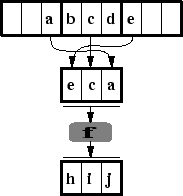The transition function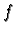now is a function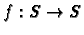, where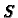is the complete state of a cell, which is assembled from the parts of the different neighbors. The complete updating step consists of two parts: assembling the partitions from the neighborhood and then application of. The first part is clearly reversible, since it is globally a permutation of the parts of the cells (assuming the boundary conditions are set correctly). If the second part is also reversible, i.e., ifis a permutation, then the whole CA is reversible by simply reversingand exchanging the order of the two substeps.

This concept of partitioned CA is also used in the lattice gas cellular automata, even for cases that are not reversible. In this case the functionis not reversible, or it is probabilistic. Nevertheless, the partitioning in this case helps ensure the conservation of mass (particle numbers): The partitioning step conserves particles (here: bits) by definition, and the functionis constructed to do so, too.

Partitioned CA can be constructed for any dimension, lattice geometry, and neighborhood size. The state set is a product set ofsets, whereis the size of the neighborhood. The parts of the state need not be equal in size.

It is trivial to see that partitioned CA are real CA in the strict sense of the definition, since they are just a restricted subset. On the other hand, normal CA can be simulated by partitioned CA by expanding the state set, and just replicating the state of a cell in each part, so that each neighbor sees a replicated version of the cell's state.

As an example, we can use a partitioned CA to simulate a one-dimensional excitable medium. Of course, the excitable medium is by its nature irreversible, so we use an irreversible function. Figure 2.20 shows the functionwe use, together with an example evolution. In the evolution, both the partitioning step and the function application are shown. The function is constructed to show left- and right moving waves, which annihilate each other when they meet.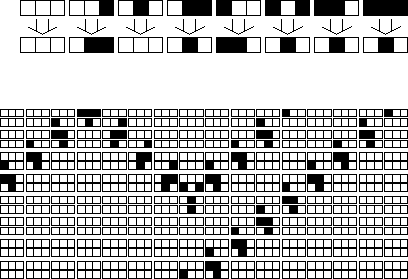A well-known class of partitioned CA are the Lattice Gas Automata (LGA).

## 2.7.2 Block Cellular Automata

We have seen that partitioned CA are an easy way to construct reversible CA or irreversible CA that obey certain conservation laws. But are they efficient?

One problem is that the state set is relatively large. A partitioned CA withneighbors has to use at leastbits, i.e.,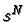states. Therefore we cannot construct a partitioned CA with only two states.

The block CA  provides an alternative: Partition the cells of a CA into a regular pattern of blocks that cover the whole space and do not overlap. Then apply a transformation function to each block synchronously and independently. This provides interaction of the cells within a block. But how do cells communicate across block boundaries? Simply shift the block boundaries for the next step. In this way the new block spans the block boundary of the precious step. Figure 2.21 illustrates this with a one-dimensional CA.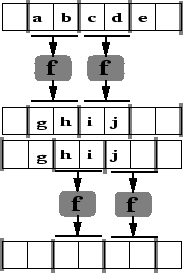In block CA the local updating function is a function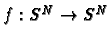. Again, the construction of a reversible CA relies on the reversibility of the partitioning and of. The partitioning does not change or even move any bits, and therefore it is reversible. If the functionis a permutation, it is also reversible and so the block CA is reversible. It is also simple to construct irreversible CA with conservation laws using the concept of block CA.

Are block CA real CA? Normal CA have state transition function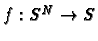and not. Nevertheless it is possible to simulate block CA with ordinary CA (by collecting all states of a block within one cell) and vice versa, so block CA are equivalent to ordinary CA. Of course this theoretical equivalence does not say much about the practicality of the different approaches. Block CA are very useful in situations where the state of a cell should be only one bit, but a (partial) conservation law holds. They can also be used in situations where a strong exclusion principle is combined with a conservation law.Next: 2.8 Summary of construction Up: 2. Choices in the Previous: 2.6 Transition rule   Contents
Jörg R. Weimar